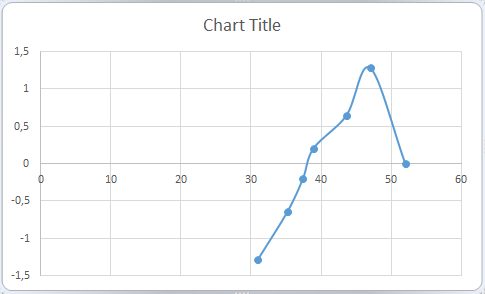#### How to Make Normal Probability Plot in Excel

You will learn how to do mathematical calculations and how to insert a probability chart (to be exact, it is a normal distribution probability plot).

Probably, it is the Excel tutorial that solves your issue.

A normal probability plot, also known as a Q-Q plot, is a graphical tool used to assess whether a set of data is approximately normally distributed. In Excel, you can create a normal probability plot using the following steps:

## Data preparation

First, you need data that looks like this: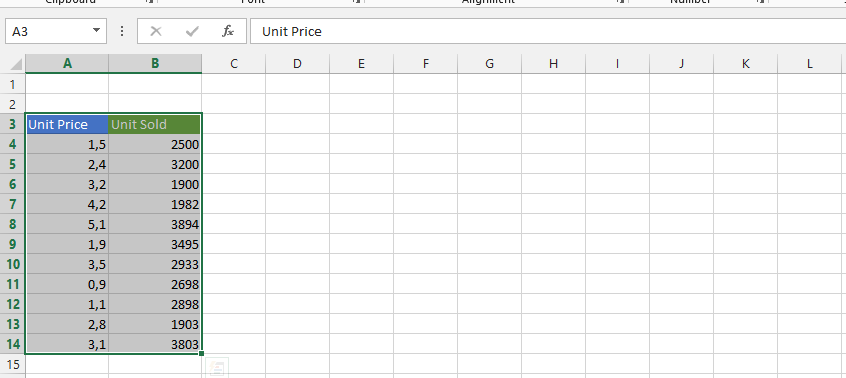Select the Home tab.Click on a value in the data, and Sort & filter to choose Sort from smallest to largest.Start another column, with one in between, and label it “i”.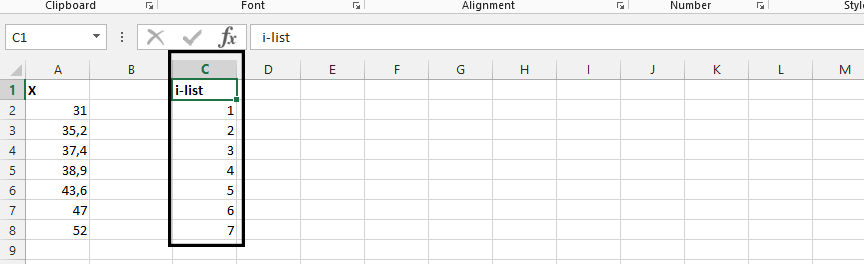Start another column, and label it formula.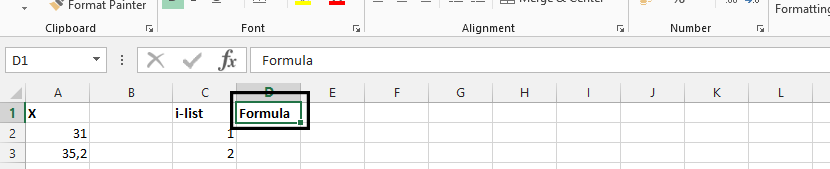Click on D2 (1), and type =(C2-0,375)/(6+0,25)(2), and press enter.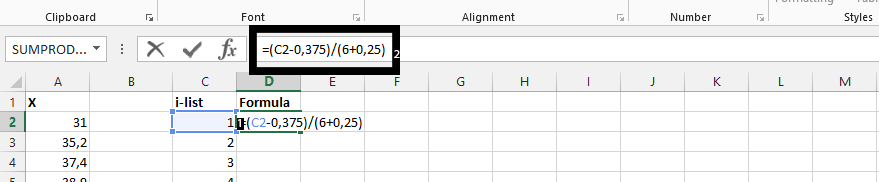Double click on the small square in the right bottom corner of the cell.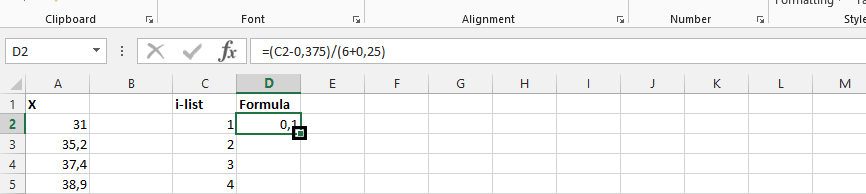Label Column B as the Expected Z.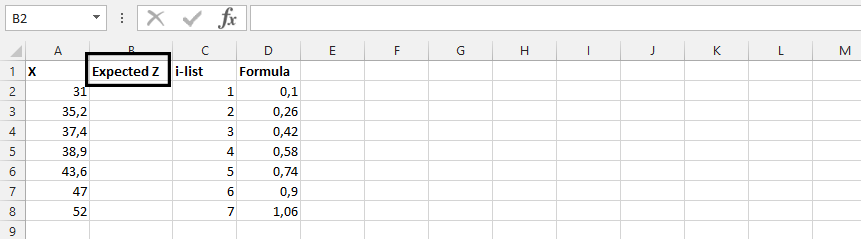## NORM.S.INV formula

Click on B2 (1), and type =NORM.S.INV(D2) (2)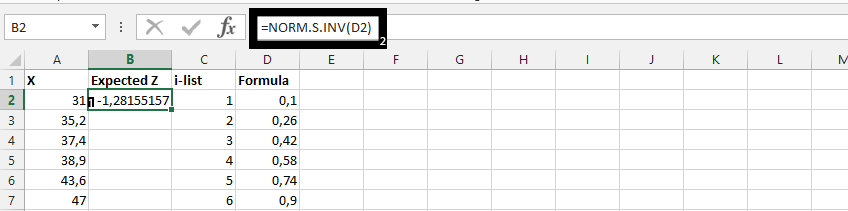Double click on the small square shown in the result from the previous step.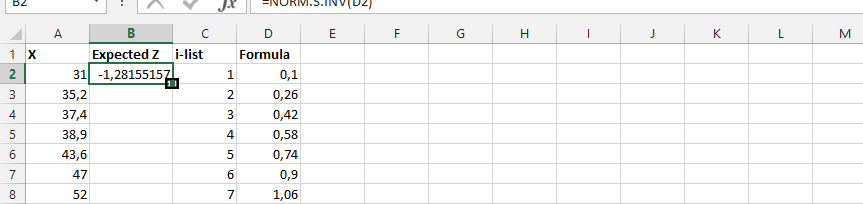## Inserting a normal distribution plot

Highlight A2-B8 (1), click on insert (2), scatter chart (3), and choose the desired chart (4) to plot the normal distribution chart.